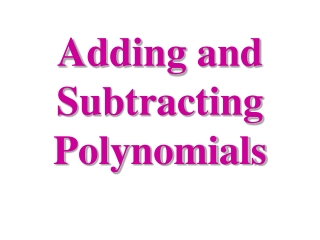DownloadDownload PresentationTélécharger la présentation- - - - - - - - - - - - - - - - - - - - - - - - - - - E N D - - - - - - - - - - - - - - - - - - - - - - - - - - -
Presentation Transcript

2. Like Terms Like Terms refers to monomials that have the same variable(s) but may have different coefficients. The variables in the terms must have the same powers. Which terms are like? 3a2b, 4ab2, 3ab, -5ab2 4ab2 and -5ab2 are like. Even though the others have the same variables, the exponents are not the same. 3a2b = 3aab, which is different from 4ab2 = 4abb.

3. Like Terms Constants are like terms. Which terms are like? 2x, -3, 5b, 0 -3 and 0 are like. Which terms are like? 3x, 2x2, 4, x 3x and x are like. Which terms are like? 2wx, w, 3x, 4xw 2wx and 4xw are like.

4. Adding Polynomials Add: (x2 + 3x + 1) + (4x2 +5) Step 1: Underline like terms: (x2 + 3x + 1) + (4x2 +5) Notice: ‘3x’ doesn’t have a like term. Step 2: Add the coefficients of like terms, do not change the powers of the variables: (x2 + 4x2) + 3x + (1 + 5) 5x2 + 3x + 6

5. Adding Polynomials Some people prefer to add polynomials by stacking them. If you choose to do this, be sure to line up the like terms! (x2 + 3x + 1) + (4x2 +5) (x2 + 3x + 1) + (4x2 +5) 5x2 + 3x + 6 Stack and add these polynomials: (2a2+3ab+4b2) + (7a2+ab+-2b2) (2a2 + 3ab + 4b2) + (7a2 + ab + -2b2) (2a2+3ab+4b2) + (7a2+ab+-2b2) 9a2 + 4ab + 2b2

6. Adding Polynomials • Add the following polynomials; you may stack them if you prefer:

7. Problem situations in Polynomials An electronics store sells cell phones and pagers. The equations below represent the monthly sales m of cell phones C and pagers P. Write the equation that represents the total monthly sales T sales of wireless devices. Use the equation to predict the number of wireless devices sold in 10 months. C = 7m + 137 P = 4m + 78

8. Subtracting Polynomials Subtract: (3x2 + 2x + 7) - (x2 + x + 4) Step 1: Change subtraction to addition (Keep-Change-Change.). (3x2 + 2x + 7) + (- x2 + - x + - 4) Step 2: Underline OR line up the like terms and add. (3x2 + 2x + 7) + (- x2 + - x + - 4) 2x2 + x + 3

9. Subtracting Polynomials • Subtract the following polynomials by changing to addition (Keep-Change-Change.), then add:

10. Problem situations in Polynomials The measures of two sides of a triangle are given. If P is the perimeter, find the measure of the third side. Sides: 2x + 3y and x – 2y P = 7x + 3y